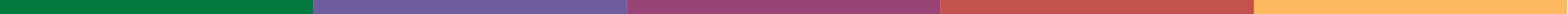# Tile Calculator

## How to calculate how many tiles you need to buy

Do you need to convert feet to metres first? Use our feet to metres converter below.

1. Firstly you need to enter the width of the tile in millimetres, for example, if the width of the tile is 150mm (6 inch), you would enter 150, enter your figure in the tile width field in the calculator below. Do the same for the tile height.
2. Next measure the width of the area to be tiled, for example, if the width of the walls are 3 metres wide you would enter 3, enter your figure in the area width field in the calculator below. Do the same for the area to be tiled height.
3. Finally, specify the percentage you wish to add for breakages and wastage, we would recommend adding 10%. Select your percentage for wastage / breakage in the calculator below and then hit the calculate button to find out how many tiles you will require.
Tile calculator
Tile size (millimetres)

Tiled area size (metres)

Breakage and wastage allowance (optional)

## Convert Feet to Metres converter

If you need to firstly convert feet to metres input you rounded up (e.g. 8 not 7'6) measurement into the feet box below and press the convert button. You can then use the resulting figures in metres in the tile calculator.

Feet to Metres converter

Disclaimer: This calculator should be used as an approximate guide only. Calculations are based on the figures you provide. We can take no responsibility for over or under ordering. We also recommend adding at least 10% for breakages and wastage.

## What people are saying about our tile calculator

“Best calculator we have seen thanks” :: “The best calculator by far” :: “Very good,and helpful” :: “Nice n easy to understand and use Thank you.” :: “Very simple and clear” :: “Great calculator, just what i needed thanks” :: “Tile calculator was great. I had been pondering how to quickly work out the required number of tiles.” :: “Thanks You, what a great calculator AAAAA*****” :: “Great, Calculated tiles required in minutes”